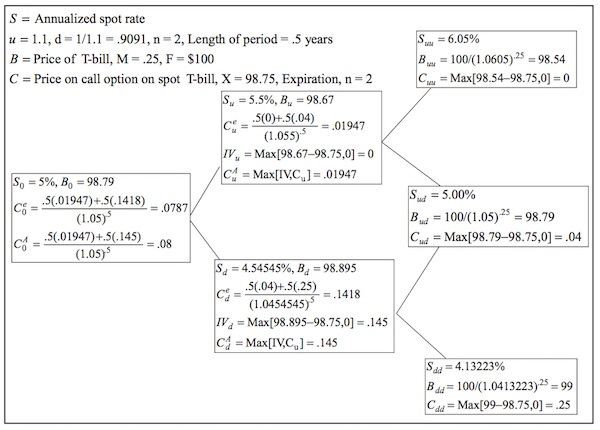### Seeing is believing!

Before you order, simply sign up for a free user account and in seconds you'll be experiencing the best in CFA exam preparation.

##### Subject 3. Interest Rate Options
The binomial interest rate tree can be used to price interest rate options. We use the expectations approach discussed before. The underlying instrument for interest rate options is the spot rate.

The figure below shows a two-period binomial tree for an annualized risk-free spot rate (S) and the corresponding prices on a T-bill (B) with a maturity of .25 years and face value of \$100. The length of each period is six months (six-month steps); the upward parameter on the spot rate (u) is 1.1 and the downward parameter (d) is 1/1.1 = 0.9091; the probability of the spot rate increasing in each period is .5; and the yield curve is assumed flat.As shown in the figure, given an initial spot rate of 5% (annual), the two possible spot rates after one period (six months) are 5.5% and 4.54545%, and the three possible rates after two periods (one year) are 6.05%, 5%, and 4.13223%. At the current spot rate of 5%, the price of the T-bill is B0 = 98.79 [= 100/(1.05).25]; in period 1, the price is 98.67 when the spot rate is 5.5% [= 100/(1.055).25] and 98.895 when the rate is 4.54545% [= 100/(1.0454545).25]. In period 2, the T-bill prices are 98.54, 98.79, and 99 for spot rates of 6.05%, 5%, and 4.13223%, respectively.

Given the binomial tree of spot rates and prices on the spot T-bill, we can determine the values of call and put options on spot T-bills.

• For European options, the methodology for determining the price is to start at expiration, where we know the possible option values are equal to their intrinsic values, IVs. Given the option's IVs at expiration, we then move to the preceding period and price the option to equal the present value of its expected cash flows for the next period. Given these values, we roll the tree to the next preceding period and again price the option to equal the present value of its expected cash flows. We continue this recursive process to the current period.
• If the option is American, then its early exercise advantage needs to be taken into account by determining at each node whether it is more valuable to hold the option or exercise it. This is done by starting one period prior to the expiration of the option and constraining the price of the option to the maximum of its binomial value (present value of next period's expected cash flows) or intrinsic value (i.e., the value from exercising). Those values are then rolled to the next preceding period, and the American option values for that period are obtained by again constraining the option prices to be the maximum of the binomial value or the IV; this process continues to the current period.

Suppose we want to value a European call on a spot T-bill with an exercise price of \$98.75 per \$100 face value and expiration of one year. To value the call option on the T-bill, we start at the option's expiration, where we know the possible call values are equal to their intrinsic values, IVs. In this case, at spot rates of 5% and 4.13223%, the call is in the money with IVs of .04 and .25, respectively, and at the spot rate of 6.05% the call is out of the money and thus has an IV of zero.

Given the three possible option values at expiration, we next move to period 1 and price the option at the two possible spot rates of 5.5% and 4.54545% to equal the present values of their expected cash flows next period. Assuming there is an equal probability of the spot rate increasing or decreasing in one period (q = .5), the two possible call values in period 1 are .01947 and .1418:

Cu = (.5 * 0 + .5 * .04)/(1.055)0.5 = .01947

Cd = (.5 * .04 + .5 * .25)/(1.0454545)0.5 = .1418
Rolling these call values to the current period and again determining the option's price as the present value of the expected cash flow, we obtain a price on the European T-bill call of .0787: C0 = (.5 * .01947 + .5 * .1418)/(1.05)0.5 = .0787.

If the call option were American, its two possible prices in period 1 are constrained to the maximum of the binomial value (present value of next period's expected cash flows) or the intrinsic value (i.e., the value from exercising): CtA = max[Ct, IV].

In period 1, the IV slightly exceeds the binomial value when the spot rate is 4.54545%. As a result, the American call price is equal to its IV of .145. Rolling this price and the upper rate's price of .01947 to the current period yields a price for the American T-bill call of .08. This price slightly exceeds the European value of .0787, reflecting the early exercise advantage of the American option.